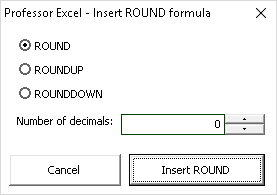# ROUND: How to Round Values in Excel (+Tip&Tricks)

In this article, talk about a basic topic in Excel: Rounding numbers. Excel provides three different versions of formulas for rounding number: ROUND, ROUNDUP and ROUNDDOWN. ROUNDUP and ROUNDDOWN always round numbers away or towards zero. In this article we take a closer look at the ROUND formula and explore advanced ways of applying it.

## When to use the ROUND formula

Excel provides three slightly different versions of formulas for rounding numbers: ROUND, ROUNDUP and ROUNDDOWN. ROUND always rounds a number to a specified number of digits. With ROUNDUP and ROUNDDOWN you can furthermore define if you want to round away from 0 or towards 0.

There are some cases in which you should use the these formulas and some cases in which you should not. First of all: Coming with the ROUND formula, your calcualtion usually lose precision. Therefore, we have to differentiate between the displayed precision and the actual precision of the underlying numbers. If you just want to display values with a certain number of digits, you should do this within the cell formatting (right click on a cell and then click on ‘Format Cells’).

But there are also some cases in which you should consider the ROUND formulas:

• If Excel calculates wrong. If Excel shows an obvious wrong result (although only a tiny deviation), you can fix this with the ROUND formula. Please refer to this article for more information about wrong results in Excel.
• For special application as shown in the examples below (e.g. classify data).

## How to use the function

The ROUND formula is quite easy to use as it has only two parts (the numbers are corresponding to the picture):

1. The value which should be rounded. Instead of a value, you can also use a cell reference or the actual calculation.
2. The number of digits you want to get your value rounded to. In the above example it’s two digits. The result is shown in cell C10.

Let’s say we want to get a number rounded to thousands. It basically works the same way, but for the number of digits, we type -3. Now our value will be rounded to the to the nearest multiple of 1,000.

ROUNDDOWN and ROUNDUP work in a similar way. ROUNDUP rounds a number up, away from 0. ROUNDDOWN rounds it towards 0. They also have two arguments: The value which is supposed to be rounded up or down as well as the number of digits.

## Tips & tricks with the ROUND formula

The ROUND formula can be used for more advanced functions than simply rounding. Let’s take a look at two more examples: Rounding to 0 or 5 and how to put values into even distributed classes.

### Round to 0 or 5

Oftentimes, you don’t just want to round to a certain number of digits. Instead, you want to get a 0 or 5 as the last digit. The approach is quite simple: We multiply our value by 2. Then we round it and afterwards divide it by 2.

Let’s say we got a our numeric value in cell A1. The formula for getting a 0 or 5 in the end looks like this:

```=ROUND(A1*2,-1)/2
```

For different numbers in cell A1, the formula will conduct the following steps:

• If A1 is 23: 23 x 2 = 46. 46 will be rounded up to 50. Afterwards, we divide by 2 so the result is 25.
• If A1 is 12: 12 x 2 = 24. 24 will be rounded down to 20. After dividing by 2 the result is 10.

### Put values into even distributed classes

Another example for advanced uses of the ROUND formulas is the classification of values. The class width is 3. So every value between 0 and 3 should be classified as 0, each value between 3 and 6 as 3 and so on. This example is shown on the picture on the right hand side.

The class width is given in cell B2, the values are shown in the table below. As the class width is 3, we divide our original values by 3. Then we use the ROUNDDOWN formula to no digits and multiply everything by the class width again.

`=ROUNDDOWN(A6/\$B\$2,0)*\$B\$2`

Again, let’s see what happens for different values in cell A6:

• So what happens, if the value in A6 is 2? 2 divided by 3 is 0.67. Rounded down to no digit: 0. 0 x 3 is still 0.
• If A6 is 5: 5 divided by 3 is 1.67, rounded down is 1. 1 x 3 is 3.

Please note, that this approach only works for even distributed classes. If you want to classify data into uneven classes, you have to choose different approaches (e.g. support tables and VLOOKUP or INDEX/MATCH).

## Expert tip: Easily insert ROUND formulasEasily insert the ROUND formula with ‘Professor Excel Tools’

There is an easy way to insert the ROUND, ROUNDDOWN or ROUNDUP formulas: The Excel add-in ‘Professor Excel Tools’ can help you wrapping these formulas with one click around existing formulas.

It even works on different formulas within your selected cell range. So if cell A1 has a VLOOKUP and cell A2 a SUM formula, ‘Professor Excel Tools’ still wraps the ROUND formula around those two formulas with just one click.

You can of course specify, how many digits your value should be rounded. Furthermore, this function updates existing formulas for rounding.

Free trial, no sign-up and no installation: Just click the download button below and activate the add-in within Excel.

This function is included in our Excel Add-In ‘Professor Excel Tools’

More than 35,000 users can’t be wrong.Henrik Schiffner is a freelance business consultant and software developer. He lives and works in Hamburg, Germany. Besides being an Excel enthusiast he loves photography and sports.

1.Sanjay says:
1.Henrik Schiffner says: